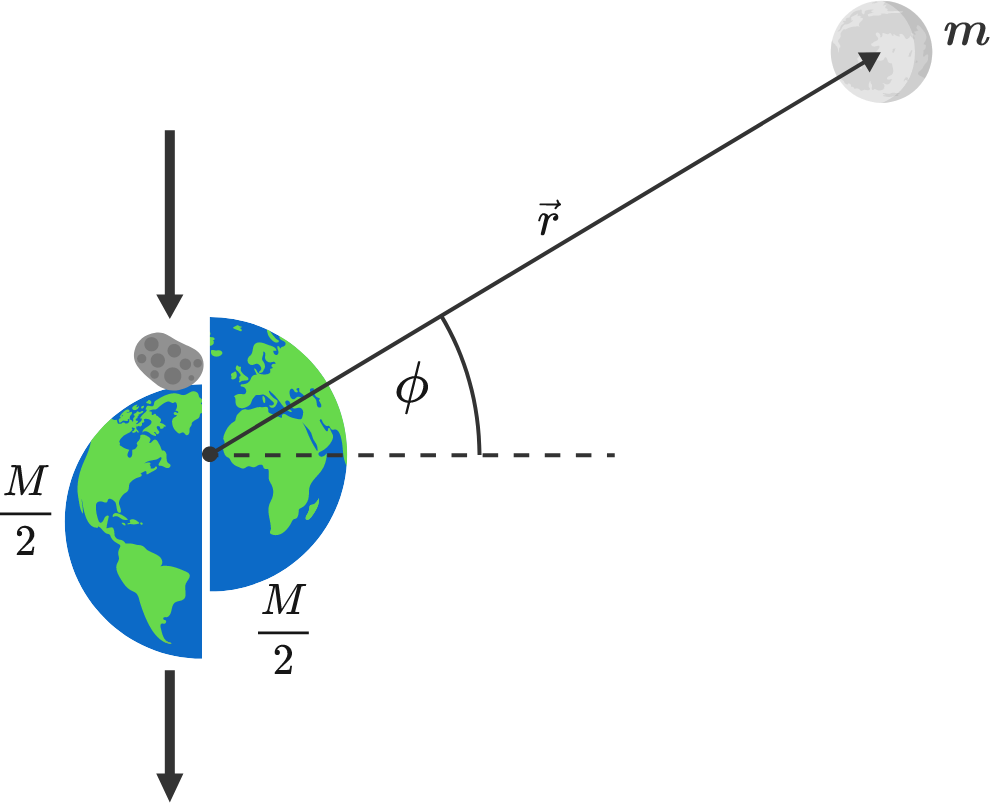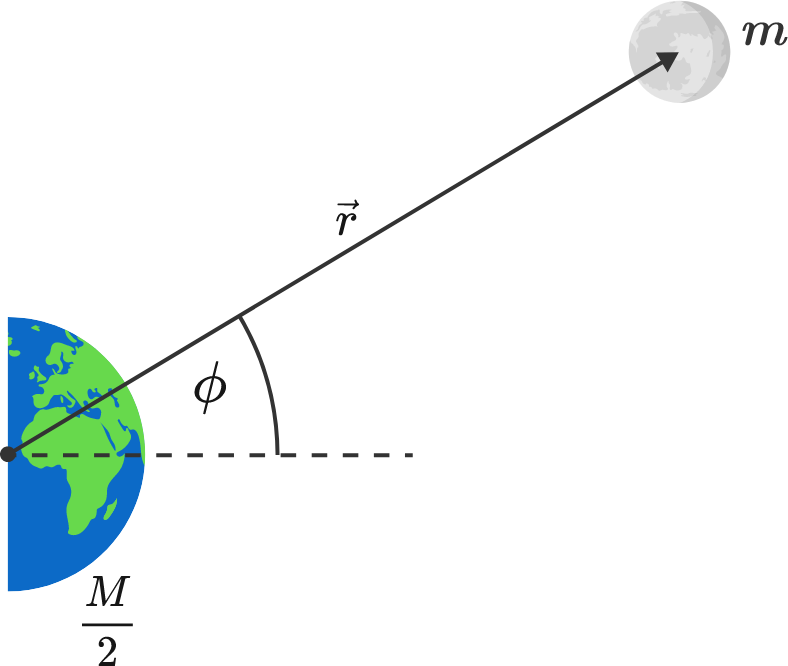# Deep impact

If half of the earth is blown away by the impact of a comet, what happens to the orbit of the moon?During impactAfter impact


Details and Assumptions:

• The mass of the earth $M$ is simply halved by the impact, without the fragments interacting with the moon of mass $m.$
• Before the impact, the moon's orbit is a perfect circle with radius $r_0.$
• $M \gg m,$ so the moon can hardly affect the motion of the earth.
• Both the energy $E = \frac{1}{2} m v^2 + V(r)$ and the angular momentum $L = m r^2 \dot \phi$ of the moon are preserved, where $r$ is an arbitrary distance between the earth and moon after the impact.
• The gravitational potential energy of the earth and moon system reads $V(r) = - G \frac{M m}{r},$ where $G$ is the gravitational constant.
×